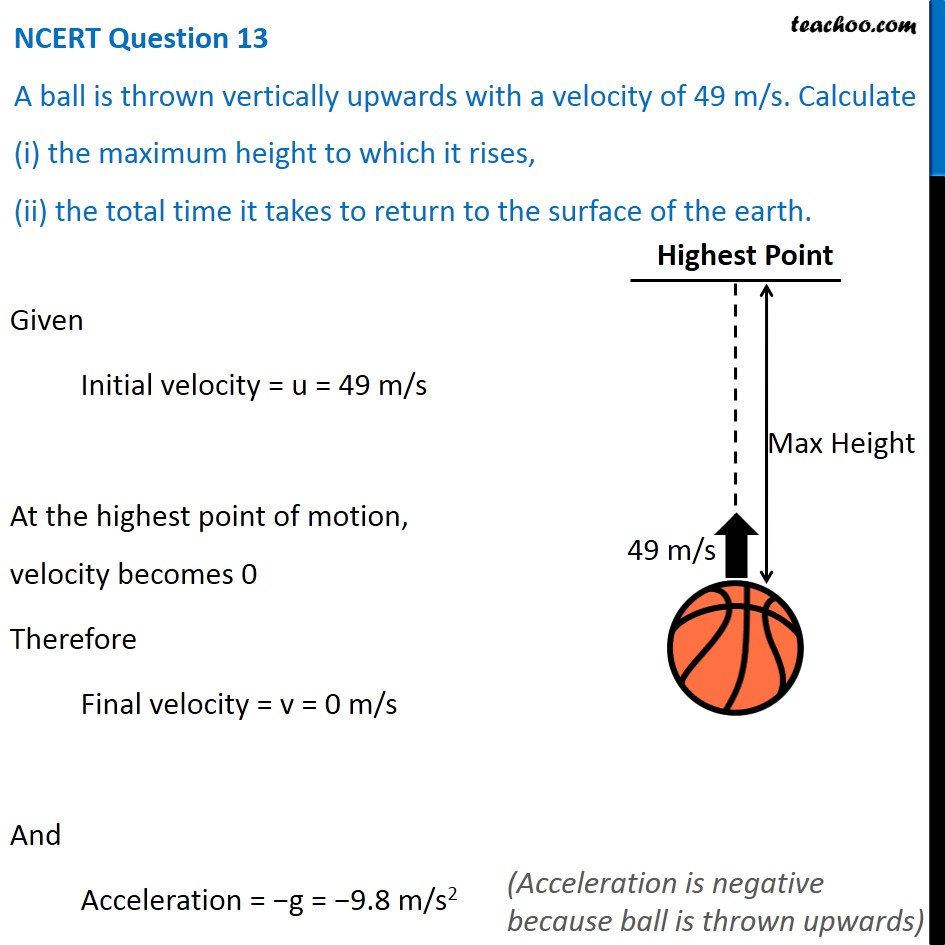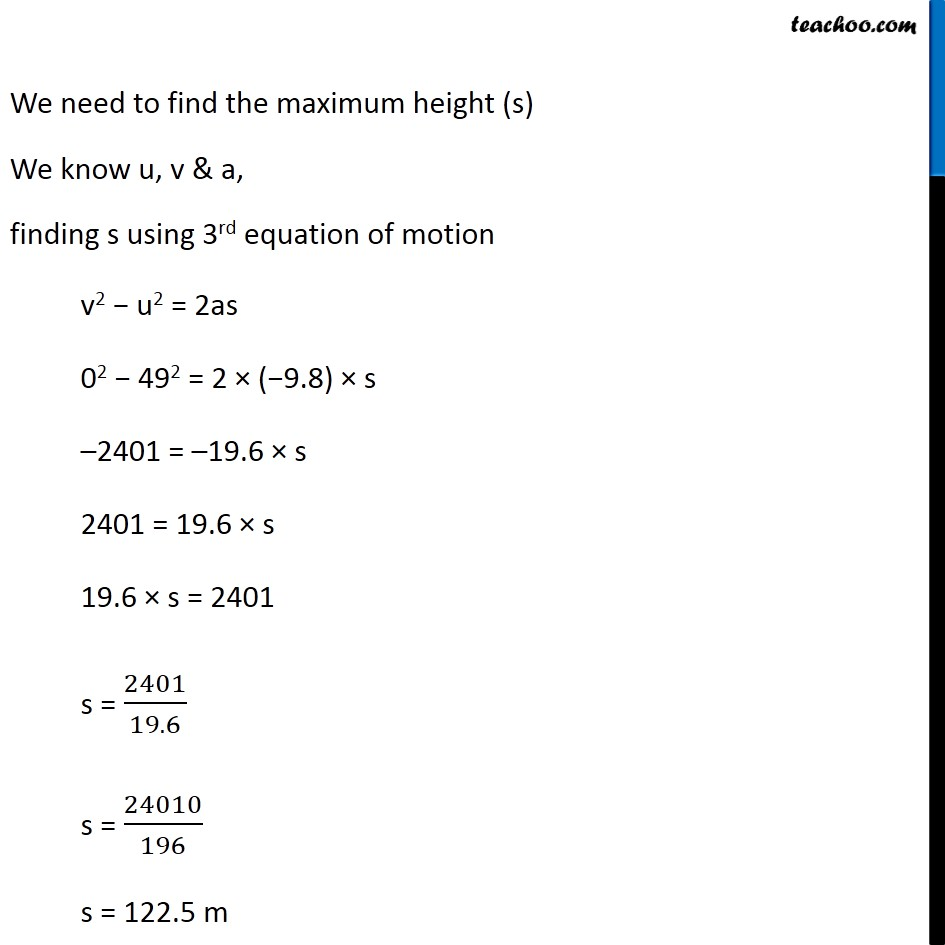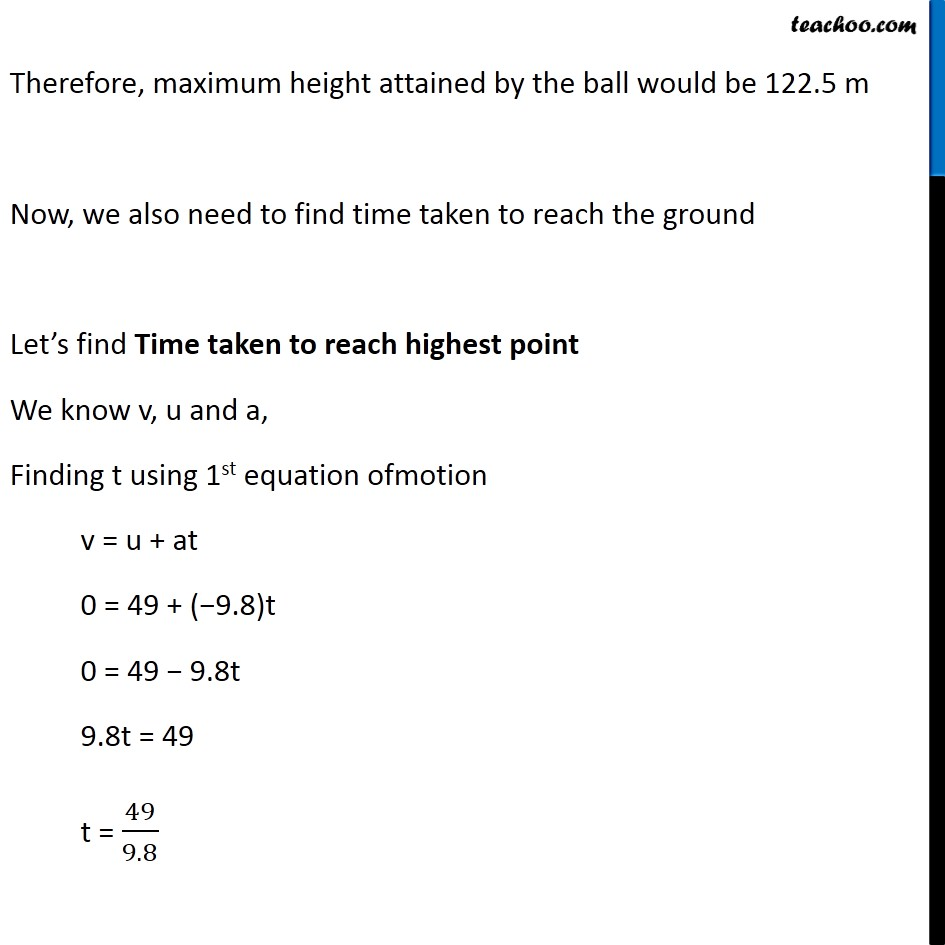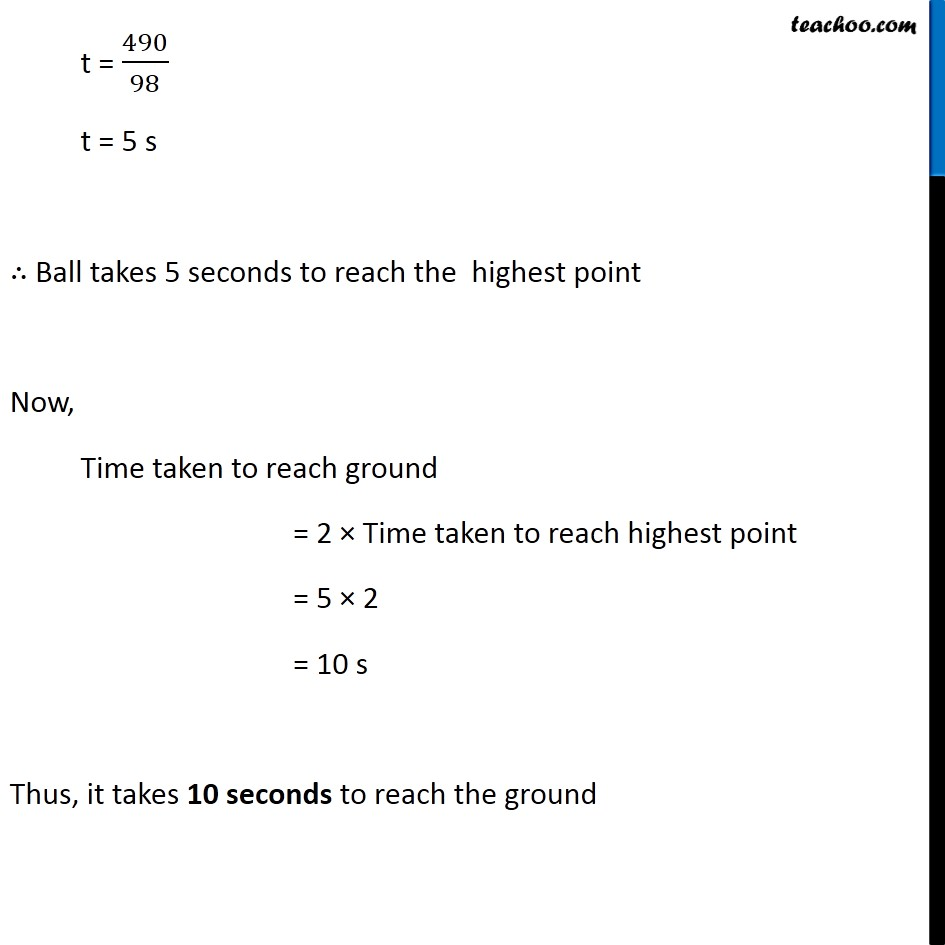NCERT Questions

Class 9
Chapter 10 Class 9 - GravitationLearn in your speed, with individual attention - Teachoo Maths 1-on-1 Class

### Transcript

NCERT Question 13 A ball is thrown vertically upwards with a velocity of 49 m/s. Calculate (i) the maximum height to which it rises, (ii) the total time it takes to return to the surface of the earth. Given Initial velocity = u = 49 m/s At the highest point of motion, velocity becomes 0 Therefore Final velocity = v = 0 m/s And Acceleration = −g = −9.8 m/s2 (Acceleration is negative because ball is thrown upwards) We need to find the maximum height (s) We know u, v & a, finding s using 3rd equation of motion v2 − u2 = 2as 02 − 492 = 2 × (−9.8) × s –2401 = –19.6 × s 2401 = 19.6 × s 19.6 × s = 2401 s = 2401/19.6 s = 24010/196 s = 122.5 m Therefore, maximum height attained by the ball would be 122.5 m Now, we also need to find time taken to reach the ground Let’s find Time taken to reach highest point We know v, u and a, Finding t using 1st equation ofmotion v = u + at 0 = 49 + (−9.8)t 0 = 49 − 9.8t 9.8t = 49 t = 49/9.8 t = 490/98 t = 5 s ∴ Ball takes 5 seconds to reach the highest point Now, Time taken to reach ground = 2 × Time taken to reach highest point = 5 × 2 = 10 s Thus, it takes 10 seconds to reach the ground